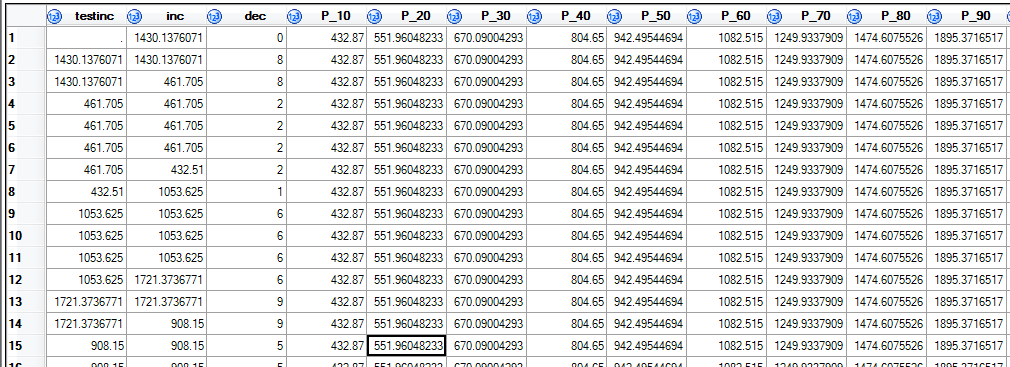## How to create groups by deciles - weird results

I'm perfoming what should be a straightwoard series of proc and data steps to categorise the 'inc' variable into deciles (pls see below code). But the result seems to be giving me a bizarre lag between one row and another, which makes no sense to me (pls see image). Please help!!!

``````*calc and output deciles;
proc univariate data=mydata noprint;
var inc;
output out=percentiles pctlpre=P_ pctlpts= 10 to 90 by 10 ;
weight myweight;
run;

*merge on deciles;
data mydata;
if _n_=1 then set percentiles;
set  mydata;
run;

*assign decile category;
data mydata;
dec=0;
if inc <P_10 then dec =1;
if P_10< inc <P_20 then dec =2;
if P_20< inc <P_30 then dec =3;
if P_30< inc <P_40 then dec =4;
if P_40< inc <P_50 then dec =5;
if P_50< inc <P_60 then dec =6;
if P_60< inc <P_70 then dec =7;
if P_70< inc <P_80 then dec =8;
if P_80< inc <P_90 then dec =9;
if P_90< inc then dec =10;
set mydata;
run;

*test the weirdness;
data mydata;
testinc=inc;
set mydata;
run;``````1 ACCEPTED SOLUTION

Accepted Solutions

## Re: How to create groups by deciles - weird results

Your last data step messes up data since set after variable assignment. Move set right after data step. Unless you wanted a lagged variable.

Otherwise, I'm not sure what's unexpected here.

Also a good example of why each data step should generate a unique data set.

Data mydata_test;;

SET my_data;

test_in = inc;

run;

4 REPLIES 4

## Re: How to create groups by deciles - weird results

Your last data step messes up data since set after variable assignment. Move set right after data step. Unless you wanted a lagged variable.

Otherwise, I'm not sure what's unexpected here.

Also a good example of why each data step should generate a unique data set.

Data mydata_test;;

SET my_data;

test_in = inc;

run;

## Re: How to create groups by deciles - weird results

Thank you, Reeza!! I see. The second last step also had set statement at the end, messing up the deciles too. Hadn't realised that it would have such an effect. Much appreciated.

## Re: How to create groups by deciles - weird results

Look at proc rank to calculate your deciles in one step.

## Re: How to create groups by deciles - weird results

Note that using IF then as you have may well not result in what you want if you have much repitition of values.

As an extreme example, if all of the data has the same value then P10=P20=P30=P40 and so forth

This example

``````data example;
input x;
datalines;
1
2
2
2
3
3
3
4
4
4
;
run;

proc univariate data=example;
var x;
run;``````

Shows that 4 would be at least P75, P80 P90 and P100. Your IF/then/else code will assign all values of 4 to the lowest percentile and none to the higher. If that is not the desired result then @Reeza's suggestion is much better.

Discussion stats
• 4 replies
• 1117 views
• 0 likes
• 3 in conversation